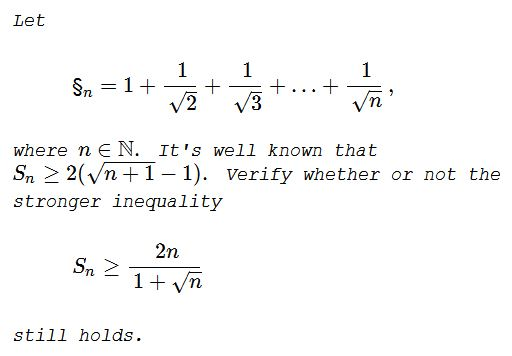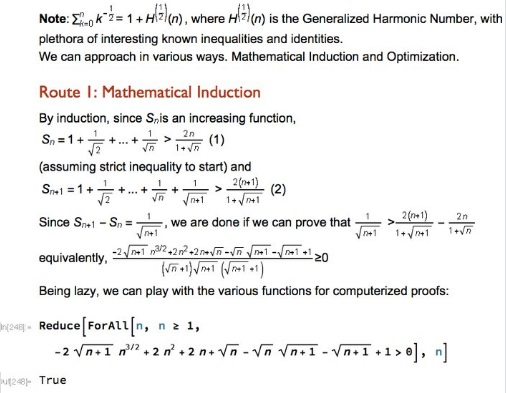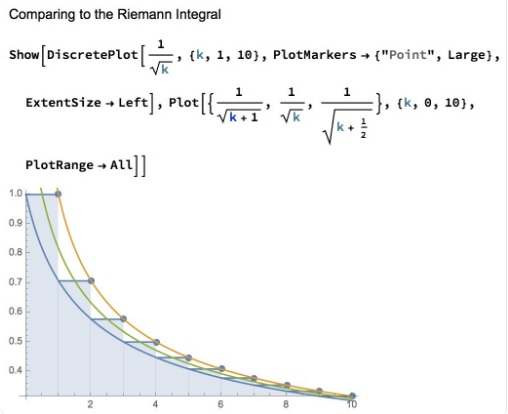# Problem 3824 from Crux Mathematicorum

### Statement### Remark

Far as the problem is concerned, there is no need to mention a weaker result. But, of cause, the weakness claim can be easily checked:

$\displaystyle 2(\sqrt{n+1}-1)=\frac{2[(n+1)-1]}{\sqrt{n+1}+1}=\frac{2n}{1+\sqrt{n+1}}\lt\frac{2n}{1+\sqrt{n}}.$

### Solution 1

Define function $\displaystyle f(x)=\frac{2x}{1+\sqrt{x}}.\;$ $\displaystyle f'(x)=\frac{2+\sqrt{x}}{(1+\sqrt{x})^2}\gt 0\;$ and $\displaystyle f''(x)=-\frac{3+\sqrt{x}}{2\sqrt{x}(1+\sqrt{x})^3}\lt 0,\;$ implying that the function is concave and strictly increasing. This, in particular, means that $f(x+1)-f(x)\lt f'(x).\;$ We are led to verify that

$\displaystyle \frac{2+\sqrt{n}}{(1+\sqrt{n})^2}\lt\frac{1}{\sqrt{n+1}}\;$ $(=S_{n+1}-S_{n}).$

Observe that $2\le\sqrt{n+1},\;$ for $n\ge 3,\;$ and the inequality is strict for $n\gt 3.\;$ Let's continue:

$2+\sqrt{n}\le\sqrt{n+1}+\sqrt{n},\\ \displaystyle\frac{2+\sqrt{n}}{\sqrt{n+1}+\sqrt{n}}\le 1,\\ (2+\sqrt{n})(\sqrt{n+1}-\sqrt{n})\le 1,\\ (2+\sqrt{n})\sqrt{n+1}\le 1+\sqrt{n}(2+\sqrt{n}),\\ (2+\sqrt{n})\sqrt{n+1}\le 1+2\sqrt{n}+n,\\ (2+\sqrt{n})\sqrt{n+1}\le (1+\sqrt{n})^2,\\ \displaystyle\frac{2+\sqrt{n}}{(1+\sqrt{n})^2}\le\frac{1}{\sqrt{n+1}}.$

Thus, $f(n+1)-f(n)\le S_{n+1}-S_{n},\;$ for $n\ge 3,\;$ and the inequality is strict for $n\gt 3.\;$ The telescoping gives $f(n+1)-f(3)\lt S_{n+1}-S_3.\;$ What remains is to show that $f(3)\lt S_3,\;$ i.e., that $\displaystyle\frac{2\cdot 6}{1+\sqrt{3}}\lt 1+\frac{1}{\sqrt{2}}+\frac{1}{\sqrt{3}}.$ We have a sequence of equivaleny inequalties:

$\displaystyle\frac{2\cdot 6}{1+\sqrt{3}}\lt 1+\frac{1}{\sqrt{2}}+\frac{1}{\sqrt{3}},\\ \displaystyle 3(\sqrt{3}-1)\lt 1+\frac{\sqrt{2}}{2}+\frac{\sqrt{3}}{3},\\ 16\sqrt{3}\lt 24+3\sqrt{2},\\ 768\lt 594+144\sqrt{2},\\ \displaystyle\frac{174}{144}\lt\sqrt{2}.$

The latter is indeed correct since $\displaystyle\frac{174}{144}\approx 1.2,\;$ while $\sqrt{2}\approx 1.4.\;$ Adding $f(n+1)-f(3)\lt S_{n+1}-S_3\;$ and $f(3)\lt S_3\;$ gives $f(n+1)\lt S_{n+1},\;$ for $n\ge 3,\;$ and as we just saw it is in fact true for $n\ge 2.\;$ Thus, we can say that $f(n)\lt S_{n},\;$ for $n\ge 3.\;$ Note that $f(1)=S_1,\;$ leaving $n=2\;$ unaccounted for. Let's check that $f(2)\lt S_2,\;$ i.e., $\displaystyle\frac{4}{1+\sqrt{2}}\lt 1+\frac{1}{\sqrt{2}}.\;$ This is equivalent to $4\sqrt{2}\lt (1+\sqrt{2})^2\;$ and, subsequently, to $2\sqrt{2}\lt 3,\;$ which is true.

So, finally, $f(n)\lt S_n,\;$ for $n\gt 1\;$ and $f(1)=S_1.$

### Solution 2### Solution 3### Acknowledgment

This is Problem 3824 from Crux Mathematicorum, proposed by Edward T. H. Wang and Dexter Wei (Waterloo, Canada). I am grateful to Marian Dinca who communicated to me the problem, along with his solution.

### Telescoping situations

• Leibniz and Pascal Triangles
• Infinite Sums and Products
• Sum of an infinite series
• Harmonic Series And Its Parts
• A Telescoping Series
• An Inequality With an Infinite Series
• That Divergent Harmonic Series
• An Elementary Proof for Euler's Series
• $\sin 1^{\circ}+\sin {2^\circ}+\sin 3^{\circ}+\cdots+\sin 180^{\circ}=\tan 89.5^{\circ}$
• $x_n=\sin 1+\sin 3+\sin 5+\cdots+\sin (2n-1)$
• A Welcome Problem for the Year 2018
•Copyright © 1996-2018 Alexander Bogomolny

 65264572